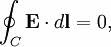### Isto é que é um verdadeiro desafio para Engenheiros

Tendo em conta estas duas Leis de Korchhoff (que não me atrevo a traduzir)...

"Kirchhoff's first law, Kirchhoff's point rule, Kirchhoff's junction rule: The principle of conservation of electric charge implies that at any point in an electrical circuit where charge density is not changing in time, the sum of currents flowing towards that point is equal to the sum of currents flowing away from that point.Kirchhoff's second law, Kirchhoff's loop rule: It is a consequence of the principle of conservation of energy. The principle of conservation of energy implies that the directed sum of the electrical potential differences around a closed circuit must be zero (otherwise, it would be possible to build a perpetual motion machine that passed a current in a circle around the circuit)."avalia as seguintes imagens: